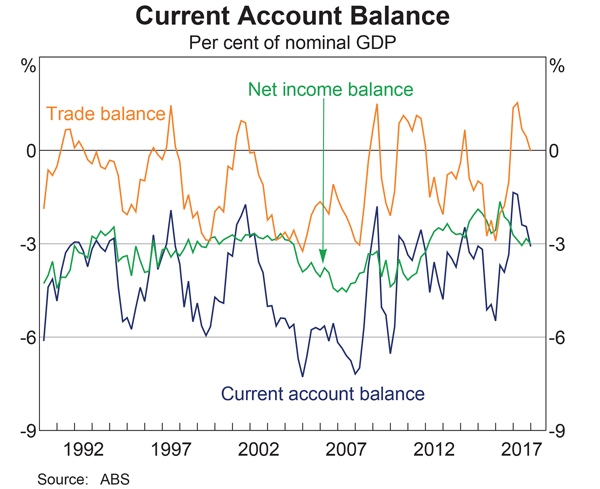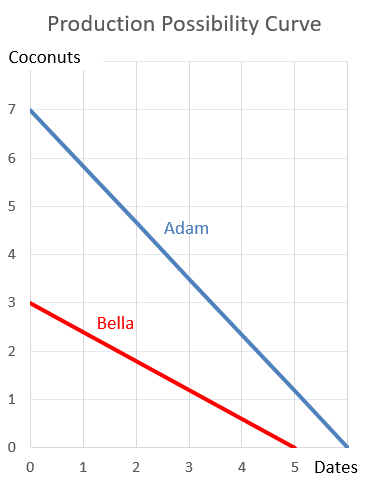# Fight Finance

#### CoursesTagsRandomAllRecentScores

The symbol $\text{GDR}_{0\rightarrow 1}$ represents a stock's gross discrete return per annum over the first year. $\text{GDR}_{0\rightarrow 1} = P_1/P_0$. The subscript indicates the time period that the return is mentioned over. So for example, $\text{AAGDR}_{1 \rightarrow 3}$ is the arithmetic average GDR measured over the two year period from years 1 to 3, but it is expressed as a per annum rate.

Which of the below statements about the arithmetic and geometric average GDR is NOT correct?

A stock has an arithmetic average continuously compounded return (AALGDR) of 10% pa, a standard deviation of continuously compounded returns (SDLGDR) of 80% pa and current stock price of $1. Assume that stock prices are log-normally distributed. In one year, what do you expect the mean and median prices to be? The answer options are given in the same order. Fred owns some Commonwealth Bank (CBA) shares. He has calculated CBA’s monthly returns for each month in the past 20 years using this formula: $$r_\text{t monthly}=\ln⁡ \left( \dfrac{P_t}{P_{t-1}} \right)$$ He then took the arithmetic average and found it to be 1% per month using this formula: $$\bar{r}_\text{monthly}= \dfrac{ \displaystyle\sum\limits_{t=1}^T{\left( r_\text{t monthly} \right)} }{T} =0.01=1\% \text{ per month}$$ He also found the standard deviation of these monthly returns which was 5% per month: $$\sigma_\text{monthly} = \dfrac{ \displaystyle\sum\limits_{t=1}^T{\left( \left( r_\text{t monthly} - \bar{r}_\text{monthly} \right)^2 \right)} }{T} =0.05=5\%\text{ per month}$$ Which of the below statements about Fred’s CBA shares is NOT correct? Assume that the past historical average return is the true population average of future expected returns. Question 984 principal agent problem, moral hazard, asymmetric information, no explanation When does the ‘principal-agent problem’ occur? Is it when: I. The principal has conflicting incentives (moral hazard); II. The agent has conflicting incentives (moral hazard); III. The principal has incomplete information about the agent (asymmetric information); or IV. The agent has incomplete information about the principal (asymmetric information)? The principal-agent problem occurs when statements: This question is about the Balance of Payments. Australia's current account as a percent of nominal gross domestic product (GDP) per annum is shown in the graph below.Assume that all foreign and domestic assets are either debt which makes interest income or equity which makes dividend income, and vice versa for liabilities which cost interest and dividend payments, respectively. Which of the following statements is NOT correct? The financing decision primarily affects which part of a business? Unrestricted negative gearing is allowed in Australia, New Zealand and Japan. Negative gearing laws allow income losses on investment properties to be deducted from a tax-payer's pre-tax personal income. Negatively geared investors benefit from this tax advantage. They also hope to benefit from capital gains which exceed the income losses. For example, a property investor buys an apartment funded by an interest only mortgage loan. Interest expense is$2,000 per month. The rental payments received from the tenant living on the property are $1,500 per month. The investor can deduct this income loss of$500 per month from his pre-tax personal income. If his personal marginal tax rate is 46.5%, this saves $232.5 per month in personal income tax. The advantage of negative gearing is an example of the benefits of: A zero coupon bond that matures in 6 months has a face value of$1,000.

The firm that issued this bond is trying to forecast its income statement for the year. It needs to calculate the interest expense of the bond this year.

The bond is highly illiquid and hasn't traded on the market. But the finance department have assessed the bond's fair value to be $950 and this is its book value right now at the start of the year. Assume that: • the firm uses the 'effective interest method' to calculate interest expense. • the market value of the bond is the same as the book value. • the firm is only interested in this bond's interest expense. Do not include the interest expense for a new bond issued to refinance the current one, as would normally happen. What will be the interest expense of the bond this year for the purpose of forecasting the income statement? A project to build a toll road will take 3 years to complete, costing three payments of$50 million, paid at the start of each year (at times 0, 1, and 2).

After completion, the toll road will yield a constant $10 million at the end of each year forever with no costs. So the first payment will be at t=4. The required return of the project is 10% pa given as an effective nominal rate. All cash flows are nominal. What is the payback period? A stock is expected to pay a dividend of$1 in one year. Its future annual dividends are expected to grow by 10% pa. So the first dividend of $1 is in one year, and the year after that the dividend will be$1.1 (=1*(1+0.1)^1), and a year later $1.21 (=1*(1+0.1)^2) and so on forever. Its required total return is 30% pa. The total required return and growth rate of dividends are given as effective annual rates. The stock is fairly priced. Calculate the pay back period of buying the stock and holding onto it forever, assuming that the dividends are received as at each time, not smoothly over each year. A firm is about to conduct a 2-for-7 rights issue with a subscription price of$10 per share. They haven’t announced the capital raising to the market yet and the share price is currently $13 per share. Assume that every shareholder will exercise their rights, the cash raised will simply be put in the bank, and the rights issue is completed so quickly that the time value of money can be ignored. Disregard signalling, taxes and agency-related effects. Which of the following statements about the rights issue is NOT correct? After the rights issue is completed: One year ago a pharmaceutical firm floated by selling its 1 million shares for$100 each. Its book and market values of equity were both $100m. Its debt totalled$50m. The required return on the firm's assets was 15%, equity 20% and debt 5% pa.

In the year since then, the firm:

• Earned net income of $29m. • Paid dividends totaling$10m.
• Discovered a valuable new drug that will lead to a massive 1,000 times increase in the firm's net income in 10 years after the research is commercialised. News of the discovery was publicly announced. The firm's systematic risk remains unchanged.

Which of the following statements is NOT correct? All statements are about current figures, not figures one year ago.

Hint: Book return on assets (ROA) and book return on equity (ROE) are ratios that accountants like to use to measure a business's past performance.

$$\text{ROA}= \dfrac{\text{Net income}}{\text{Book value of assets}}$$

$$\text{ROE}= \dfrac{\text{Net income}}{\text{Book value of equity}}$$

The required return on assets $r_V$ is a return that financiers like to use to estimate a business's future required performance which compensates them for the firm's assets' risks. If the business were to achieve realised historical returns equal to its required returns, then investment into the business's assets would have been a zero-NPV decision, which is neither good nor bad but fair.

$$r_\text{V, 0 to 1}= \dfrac{\text{Cash flow from assets}_\text{1}}{\text{Market value of assets}_\text{0}} = \dfrac{CFFA_\text{1}}{V_\text{0}}$$

Similarly for equity and debt.

One year ago you bought $100,000 of shares partly funded using a margin loan. The margin loan size was$70,000 and the other \$30,000 was your own wealth or 'equity' in the share assets.

The interest rate on the margin loan was 7.84% pa.

Over the year, the shares produced a dividend yield of 4% pa and a capital gain of 5% pa.

What was the total return on your wealth? Ignore taxes, assume that all cash flows (interest payments and dividends) were paid and received at the end of the year, and all rates above are effective annual rates.

Hint: Remember that wealth in this context is your equity (E) in the house asset (V = D+E) which is funded by the loan (D) and your deposit or equity (E).

You work for XYZ company and you’ve been asked to evaluate a new project which has double the systematic risk of the company’s other projects.

You use the Capital Asset Pricing Model (CAPM) formula and input the treasury yield $(r_f )$, market risk premium $(r_m-r_f )$ and the company’s asset beta risk factor $(\beta_{XYZ} )$ into the CAPM formula which outputs a return.

This return that you’ve just found is:

Adam and Bella are the only people on a remote island. Their production possibility curves are shown in the graph.Which of the following statements is NOT correct?